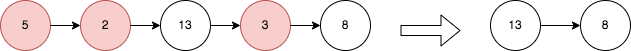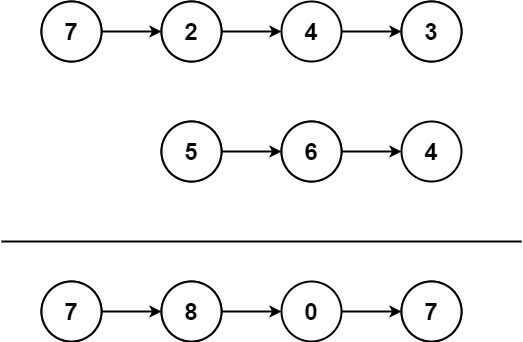2023-02-2300

1. 从链表中移除节点
1.1 题目描述
1.2 解法一：递归
1.3 迭代：反转两次链表
2. 链表中的两数相加
2.1 题目描述
2.2 解：链表反转相加
3. 链表求和
3.1 题目描述
3.2 解
4. 合并零之间的节点
4.1 题目描述
4.2 解

# 前言

1. `NO.17`2487. 从链表中移除节点
2. `NO.18`剑指 Offer II 025. 链表中的两数相加
3. `NO.19`面试题 02.05. 链表求和
4. `NO.20`2181. 合并零之间的节点

# 1. 从链表中移除节点

## 1.1 题目描述``````输入： head = [5,2,13,3,8]

- 节点 13 在节点 5 右侧。
- 节点 13 在节点 2 右侧。
- 节点 8 在节点 3 右侧。
``````

``````输入： head = [1,1,1,1]

``````

• 给定列表中的节点数目在范围 `[1, 105]` 内
• `1 <= Node.val <= 105`

## 1.2 解法一：递归

```ts```function removeNodes(head: ListNode | null): ListNode | null {
let curr: ListNode | null = head;
if (curr && !curr.next) {
return curr;
}
let newHead: ListNode | null = removeNodes(curr!.next);
} else {
}
}
``````

• 时间复杂度：`O(n)`，其中 `n` 为链表的长度。
• 空间复杂度：`O(n)`，需要 `O(n)` 的栈空间。

## 1.3 迭代：反转两次链表

```ts```// 1 -> 2 -> 3 -> 4 -> 5
// 1 <- 2 <- 3 <- 4 <- 5
function reverseList(head: ListNode | null): ListNode | null {
let prev: ListNode | null = null;
let curr: ListNode | null = head;
let next: ListNode | null = head?.next ?? null;

if (!curr || !next) return head;

while (curr && next) {
curr.next = prev;
prev = curr;
curr = next;
next = next?.next;
curr.next = prev;
}
return curr;
}

// 5 -> 2 -> 13 -> 3 -> 8
// 8 -> 3 -> 13 -> 2 -> 5
//13 -> 8
function removeNodes(head: ListNode | null): ListNode | null {
let curr: ListNode | null = newHead;
while (curr && curr.next) {
while (curr && curr.next && curr.val > curr.next.val) curr.next = curr.next.next;
curr = curr.next;
}
}
``````

• 时间复杂度：`O(n)`，其中 `n` 为链表的长度。
• 空间复杂度：`O(1)`，仅用到若干额外变量。

# 2. 链表中的两数相加

## 2.1 题目描述``````输入： l1 = [7,2,4,3], l2 = [5,6,4]

``````

``````输入： l1 = [2,4,3], l2 = [5,6,4]

``````

``````输入： l1 = , l2 = 

``````

• 链表的长度范围为` [1, 100]`
• `0 <= node.val <= 9`
• 输入数据保证链表代表的数字无前导 0

## 2.2 解：链表反转相加

```ts```function reverseList(head: ListNode | null): ListNode | null {
let prev: ListNode | null = null;
let curr: ListNode | null = head;
let next: ListNode | null = curr?.next ?? null;

while (curr && next) {
curr.next = prev;
prev = curr;
curr = next;
next = next.next;
curr.next = prev;
}
return curr;
}

l1: ListNode | null,
l2: ListNode | null
): ListNode | null {
l1 = reverseList(l1);
l2 = reverseList(l2);
let tmp: number = 0;
let ret = new ListNode();
let p = ret;

while (l1 && l2) {
p.next = new ListNode((tmp + l1.val + l2.val) % 10);
tmp = Math.floor((tmp + l1.val + l2.val) / 10);
l1 = l1.next;
l2 = l2.next;
p = p.next;
}

while (l1) {
p.next = new ListNode((tmp + l1.val) % 10);
tmp = Math.floor((tmp + l1.val) / 10);
l1 = l1.next;
p = p.next;
}

while (l2) {
p.next = new ListNode((tmp + l2.val) % 10);
tmp = Math.floor((tmp + l2.val) / 10);
l2 = l2.next;
p = p.next;
}

if (tmp) p.next = new ListNode(tmp);
return reverseList(ret.next);
}
``````

# 3. 链表求和

## 3.1 题目描述

``````输入： (7 -> 1 -> 6) + (5 -> 9 -> 2)，即617 + 295

``````

``````输入： (6 -> 1 -> 7) + (2 -> 9 -> 5)，即617 + 295

``````

## 3.2 解

```ts```function addTwoNumbers(
l1: ListNode | null,
l2: ListNode | null
): ListNode | null {
let tmp: number = 0;
let ret: ListNode | null = new ListNode();
let p = ret;
while (l1 && l2) {
p.next = new ListNode((l1.val + l2.val + tmp) % 10);
tmp = Math.floor((l1.val + l2.val + tmp) / 10);
l1 = l1.next;
l2 = l2.next;
p = p.next;
}

while (l1) {
p.next = new ListNode((l1.val + tmp) % 10);
tmp = Math.floor((l1.val + tmp) / 10);
l1 = l1.next;
p = p.next;
}

while (l2) {
p.next = new ListNode((l2.val + tmp) % 10);
tmp = Math.floor((l2.val + tmp) / 10);
l2 = l2.next;
p = p.next;
}

if (tmp) p.next = new ListNode(tmp);
return ret.next;
}
``````

# 4. 合并零之间的节点

## 4.1 题目描述

返回修改后链表的头节点 `head` 。``````输入： head = [0,3,1,0,4,5,2,0]

- 标记为绿色的节点之和：3 + 1 = 4
- 标记为红色的节点之和：4 + 5 + 2 = 11
````````````输入： head = [0,1,0,3,0,2,2,0]

- 标记为绿色的节点之和：1 = 1
- 标记为红色的节点之和：3 = 3
- 标记为黄色的节点之和：2 + 2 = 4
``````

• 列表中的节点数目在范围 `[3, 2 * 105]` 内
• `0 <= Node.val <= 1000`
•  存在连续两个 `Node.val == 0` 的节点
• 链表的 开端 和 末尾 节点都满足 `Node.val == 0`

## 4.2 解

```ts```function mergeNodes(head: ListNode | null): ListNode | null {
let curr: ListNode | null = head;
let ret: ListNode | null = new ListNode();
let p = ret;
let num: number = 0;
while(curr) {
if(curr.val === 0 && num !== 0) {
p.next = new ListNode(num)
p = p.next
num = 0
}

if(curr.val !== 0) {
num += curr.val
}
curr = curr.next
}
return ret.next
}
``````